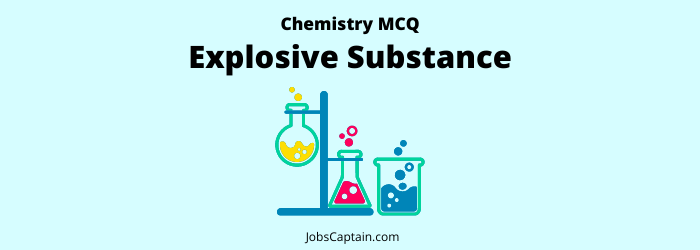# MCQ on Explosive Substance Quiz: ChemistryQuestion 1. ‘Nobel Oil’ is the name of which one of the following explosives?

(A) TNT

(B) TNA

(C) TNP

(D) TNG

(D) TNG

Question 2. RDX was invented by_______.

(A) Henning

(B) Bergillins

(C) Soddy

(D) Alfred Nobel

(A) Henning

Question 3. A mixture of which one of the following pairs of gases is the cause of occurrence of most of the explosions in mines?

(A) Carbon dioxide and Methane

(B) Methane and Air

(C) Oxygen and Acetylene

(D) Hydrogen and Oxygen

(B) Methane and Air

Question 4. Which one of the following is not an explosive?

(A) Trinitrotoluene (TNT)

(B) Cyclotrimethylene Trinitramine (RDX)

(C) Nitrochloroform

(D) Trinitroglycerine

(C) Nitrochloroform

Question 5. Which one of the following statement is correct?

(A) LSD is an antiviral compound

(B) RDX is an explosive

(C) TNT is an insecticide

(D) DDT is antibacterial compound

(B) RDX is an explosive

Question 6. Which one of the following is another name of RDX?

(A) Cyclonite

(B) Cyclohexane

(C) Dextran

(D) Cyanohydrin

(A) Cyclonite

Question 7. Which one of the following is used as an explosive?

(A) Nitroglycerine

(B) Graphite

(C) Mercuric Oxide

(D) Phosphorus Trichloride

(A) Nitroglycerine

Question 8. Which one of the following explosives does not contain nitroglycerine as an essential constituent?

(A) Amatol

(B) Dynamite

(C) Blasting Gelatine

(D) Cordite

(A) Amatol

Question 9. Which one of the following is used in the production of explosives?

(A) Oxalic acid

(B) Urea

(C) Methanol

(D) Glycerol

(D) Glycerol

Question 10. The chemical used in manufacturing of dynamite is ______.

(A) Glycerol tri-iodate

(B) Glycerol trinitrate

(C) Glycerol triacetate

(D) Glycerol